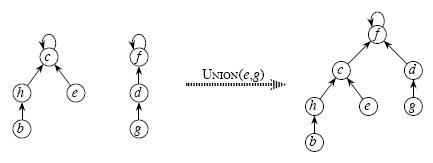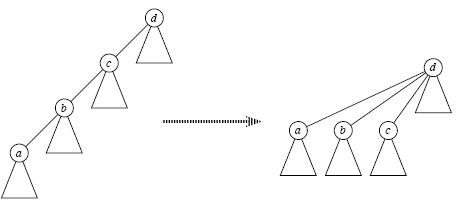# 并查集 -- 学习详解

昨天和今天学习了并查集和trie树，并练习了三道入门题目，理解更为深刻，觉得有必要总结一下，这其中的内容定义之类的是取自网络，操作的说明解释及程序的注释部分为个人理解。

并查集学习：

• 并查集：(union-find sets)

• 并查集的精髓（即它的三种操作，结合实现代码模板进行理解）：

1、Make_Set(x) 把每一个元素初始化为一个集合

2、Find_Set(x) 查找一个元素所在的集合

3、Union(x,y) 合并x,y所在的两个集合• 并查集的优化

1、Find_Set(x)时 路径压缩

2、Union(x,y)时 按秩合并• 主要代码实现
注意：
代码中路径压缩时秩不需变化的，正如Eillen所说，秩只是表示节点高度的一个上界
如果用秩进行计数，路径压缩也是不需要变化的
因为所属集合的根节点的秩在合并时已经更新，其他子节点的秩不用到也无需再变化；
int father[MAX];   /* father[x]表示x的父节点*/
int rank[MAX];     /* rank[x]表示x的秩*/

/* 初始化集合*/

void Make_Set(int x)
{
father[x] = x; //根据实际情况指定的父节点可变化
rank[x] = 0;   //根据实际情况初始化秩也有所变化
}

/* 查找x元素所在的集合,回溯时压缩路径*/

int Find_Set(int x)
{
if (x != father[x])
{
father[x] = Find_Set(father[x]); //这个回溯时的压缩路径是精华
}
return father[x];
}

/*

*/

void Union(int x, int y)
{
x = Find_Set(x);
y = Find_Set(y);
if (x == y) return;
if (rank[x] > rank[y])
{
father[y] = x;
rank[x] += rank[y];
}else
{
if (rank[x] == rank[y])
{
rank[y]++;
}
father[x] = y;
}
}

1161解题报告
2524解题报告

07-29503907-2110万+
10-121万+
10-121412
05-15852